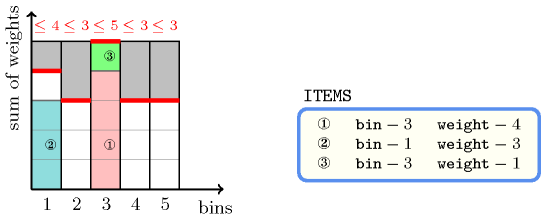## 5.54. bin_packing_capa

Origin
Constraint

$\mathrm{𝚋𝚒𝚗}_\mathrm{𝚙𝚊𝚌𝚔𝚒𝚗𝚐}_\mathrm{𝚌𝚊𝚙𝚊}\left(\mathrm{𝙱𝙸𝙽𝚂},\mathrm{𝙸𝚃𝙴𝙼𝚂}\right)$

Arguments
 $\mathrm{𝙱𝙸𝙽𝚂}$ $\mathrm{𝚌𝚘𝚕𝚕𝚎𝚌𝚝𝚒𝚘𝚗}\left(\mathrm{𝚒𝚍}-\mathrm{𝚒𝚗𝚝},\mathrm{𝚌𝚊𝚙𝚊}-\mathrm{𝚒𝚗𝚝}\right)$ $\mathrm{𝙸𝚃𝙴𝙼𝚂}$ $\mathrm{𝚌𝚘𝚕𝚕𝚎𝚌𝚝𝚒𝚘𝚗}\left(\mathrm{𝚋𝚒𝚗}-\mathrm{𝚍𝚟𝚊𝚛},\mathrm{𝚠𝚎𝚒𝚐𝚑𝚝}-\mathrm{𝚒𝚗𝚝}\right)$
Restrictions
 $|\mathrm{𝙱𝙸𝙽𝚂}|>0$ $\mathrm{𝚛𝚎𝚚𝚞𝚒𝚛𝚎𝚍}$$\left(\mathrm{𝙱𝙸𝙽𝚂},\left[\mathrm{𝚒𝚍},\mathrm{𝚌𝚊𝚙𝚊}\right]\right)$ $\mathrm{𝚍𝚒𝚜𝚝𝚒𝚗𝚌𝚝}$$\left(\mathrm{𝙱𝙸𝙽𝚂},\mathrm{𝚒𝚍}\right)$ $\mathrm{𝙱𝙸𝙽𝚂}.\mathrm{𝚒𝚍}\ge 1$ $\mathrm{𝙱𝙸𝙽𝚂}.\mathrm{𝚒𝚍}\le |\mathrm{𝙱𝙸𝙽𝚂}|$ $\mathrm{𝙱𝙸𝙽𝚂}.\mathrm{𝚌𝚊𝚙𝚊}\ge 0$ $\mathrm{𝚛𝚎𝚚𝚞𝚒𝚛𝚎𝚍}$$\left(\mathrm{𝙸𝚃𝙴𝙼𝚂},\left[\mathrm{𝚋𝚒𝚗},\mathrm{𝚠𝚎𝚒𝚐𝚑𝚝}\right]\right)$ $\mathrm{𝚒𝚗}_\mathrm{𝚊𝚝𝚝𝚛}$$\left(\mathrm{𝙸𝚃𝙴𝙼𝚂},\mathrm{𝚋𝚒𝚗},\mathrm{𝙱𝙸𝙽𝚂},\mathrm{𝚒𝚍}\right)$ $\mathrm{𝙸𝚃𝙴𝙼𝚂}.\mathrm{𝚠𝚎𝚒𝚐𝚑𝚝}\ge 0$
Purpose

Given several items of the collection $\mathrm{𝙸𝚃𝙴𝙼𝚂}$ (each of them having a specific weight), and different bins described the the items of collection $\mathrm{𝙱𝙸𝙽𝚂}$ (each of them having a specific capacity $\mathrm{𝚌𝚊𝚙𝚊}$), assign each item to a bin so that the total weight of the items in each bin does not exceed the capacity of the bin.

Example
$\left(\begin{array}{c}〈\begin{array}{cc}\mathrm{𝚒𝚍}-1\hfill & \mathrm{𝚌𝚊𝚙𝚊}-4,\hfill \\ \mathrm{𝚒𝚍}-2\hfill & \mathrm{𝚌𝚊𝚙𝚊}-3,\hfill \\ \mathrm{𝚒𝚍}-3\hfill & \mathrm{𝚌𝚊𝚙𝚊}-5,\hfill \\ \mathrm{𝚒𝚍}-4\hfill & \mathrm{𝚌𝚊𝚙𝚊}-3,\hfill \\ \mathrm{𝚒𝚍}-5\hfill & \mathrm{𝚌𝚊𝚙𝚊}-3\hfill \end{array}〉,\hfill \\ 〈\begin{array}{cc}\mathrm{𝚋𝚒𝚗}-3\hfill & \mathrm{𝚠𝚎𝚒𝚐𝚑𝚝}-4,\hfill \\ \mathrm{𝚋𝚒𝚗}-1\hfill & \mathrm{𝚠𝚎𝚒𝚐𝚑𝚝}-3,\hfill \\ \mathrm{𝚋𝚒𝚗}-3\hfill & \mathrm{𝚠𝚎𝚒𝚐𝚑𝚝}-1\hfill \end{array}〉\hfill \end{array}\right)$

The $\mathrm{𝚋𝚒𝚗}_\mathrm{𝚙𝚊𝚌𝚔𝚒𝚗𝚐}_\mathrm{𝚌𝚊𝚙𝚊}$ constraint holds since the sum of the height of items that are assigned to bins 1 and 3 is respectively equal to 3 and 5. The previous quantities are respectively less than or equal to the maximum capacities 4 and 5 of bins 1 and 3. Figure 5.54.1 shows the solution associated with the example.

##### Figure 5.54.1. Bin-packing solution to the Example slotTypical
 $|\mathrm{𝙱𝙸𝙽𝚂}|>1$ $\mathrm{𝚛𝚊𝚗𝚐𝚎}$$\left(\mathrm{𝙱𝙸𝙽𝚂}.\mathrm{𝚌𝚊𝚙𝚊}\right)>1$ $\mathrm{𝙱𝙸𝙽𝚂}.\mathrm{𝚌𝚊𝚙𝚊}>$$\mathrm{𝚖𝚊𝚡𝚟𝚊𝚕}$$\left(\mathrm{𝙸𝚃𝙴𝙼𝚂}.\mathrm{𝚠𝚎𝚒𝚐𝚑𝚝}\right)$ $\mathrm{𝙱𝙸𝙽𝚂}.\mathrm{𝚌𝚊𝚙𝚊}\le$$\mathrm{𝚜𝚞𝚖}$$\left(\mathrm{𝙸𝚃𝙴𝙼𝚂}.\mathrm{𝚠𝚎𝚒𝚐𝚑𝚝}\right)$ $|\mathrm{𝙸𝚃𝙴𝙼𝚂}|>1$ $\mathrm{𝚛𝚊𝚗𝚐𝚎}$$\left(\mathrm{𝙸𝚃𝙴𝙼𝚂}.\mathrm{𝚋𝚒𝚗}\right)>1$ $\mathrm{𝚛𝚊𝚗𝚐𝚎}$$\left(\mathrm{𝙸𝚃𝙴𝙼𝚂}.\mathrm{𝚠𝚎𝚒𝚐𝚑𝚝}\right)>1$ $\mathrm{𝙸𝚃𝙴𝙼𝚂}.\mathrm{𝚠𝚎𝚒𝚐𝚑𝚝}>0$
Symmetries
• Items of $\mathrm{𝙱𝙸𝙽𝚂}$ are permutable.

• Items of $\mathrm{𝙸𝚃𝙴𝙼𝚂}$ are permutable.

• $\mathrm{𝙱𝙸𝙽𝚂}.\mathrm{𝚌𝚊𝚙𝚊}$ can be increased.

• $\mathrm{𝙸𝚃𝙴𝙼𝚂}.\mathrm{𝚠𝚎𝚒𝚐𝚑𝚝}$ can be decreased to any value $\ge 0$.

• All occurrences of two distinct values in $\mathrm{𝙱𝙸𝙽𝚂}.\mathrm{𝚒𝚍}$ or $\mathrm{𝙸𝚃𝙴𝙼𝚂}.\mathrm{𝚋𝚒𝚗}$ can be swapped; all occurrences of a value in $\mathrm{𝙱𝙸𝙽𝚂}.\mathrm{𝚒𝚍}$ or $\mathrm{𝙸𝚃𝙴𝙼𝚂}.\mathrm{𝚋𝚒𝚗}$ can be renamed to any unused value.

Arg. properties

Contractible wrt. $\mathrm{𝙸𝚃𝙴𝙼𝚂}$.

Remark

In MiniZinc (http://www.minizinc.org/) there is also a constraint called $\mathrm{𝚋𝚒𝚗}_\mathrm{𝚙𝚊𝚌𝚔𝚒𝚗𝚐}_\mathrm{𝚕𝚘𝚊𝚍}$ which, for each bin has a domain variable that is equal to the sum of the weights assigned to the corresponding bin.

Systems
generalisation: $\mathrm{𝚒𝚗𝚍𝚎𝚡𝚎𝚍}_\mathrm{𝚜𝚞𝚖}$ (negative contribution also allowed).
specialisation: $\mathrm{𝚋𝚒𝚗}_\mathrm{𝚙𝚊𝚌𝚔𝚒𝚗𝚐}$ (non-fixed capacity replaced by fixed overall capacity).